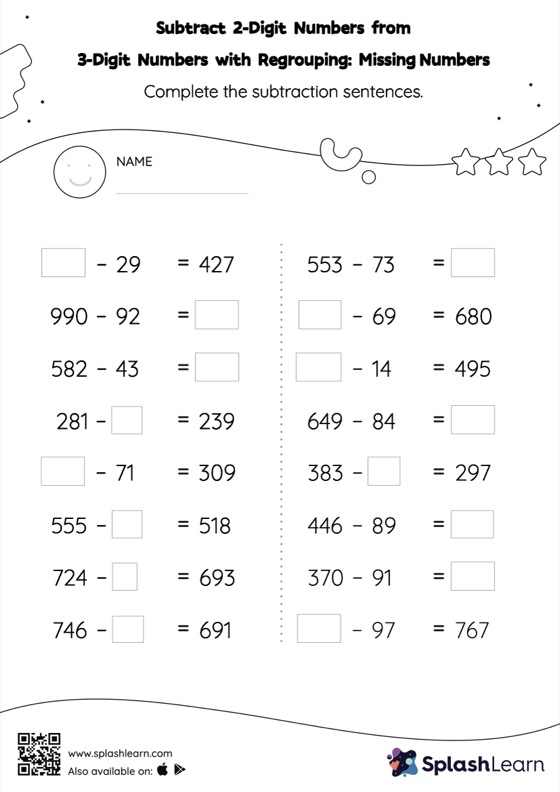# Subtract 2-Digit Numbers from 3-Digit Numbers with Regrouping: Missing Numbers Worksheet

Home > Subtract 2-Digit Numbers from 3-Digit Numbers with Regrouping: Missing NumbersHelp your little one develop a knack for math with this subtract 2-digit numbers from 3-digit numbers with regrouping worksheet. Students use the count back strategy or the relationship between addition and subtraction to find the missing number in this worksheet. They also need to regroup numbers to get to the answer in subtract 2-digit numbers from 3-digit numbers with regrouping worksheet.